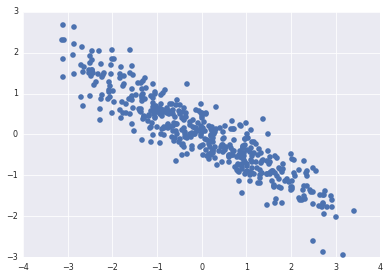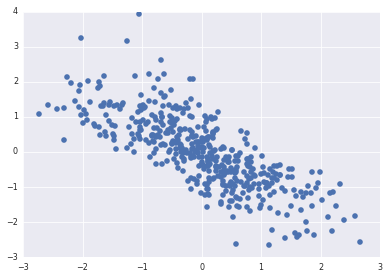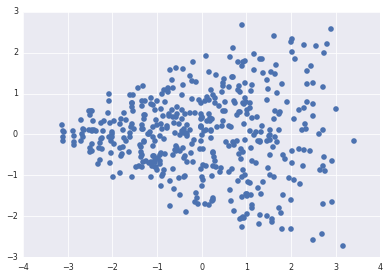In :
!date
import numpy as np, pandas as pd, pymc as pm, matplotlib.pyplot as plt, seaborn as sns
%matplotlib inline
sns.set_context('paper')
sns.set_style('darkgrid')

Sun May  3 20:21:15 PDT 2015


Each data entry has following information: Deputy name, date, bill number, vote. The vote field can be yes, no, didn't vote (treated as no) and not present.

Simulation of data extracted from this format:

In :
np.random.seed(12345) # set random seed for reproducibility

n = 450 # number of voters
p = 30 # number of votes

# latent variables for how and how often voters vote
position = np.random.normal(size=n)
activity = np.random.normal(size=n)

# simulate complete data of how voters vote
# and observed data of how/whether they vote
for i in range(n):
for j in range(p):
if np.random.rand() < np.exp(position[i]) / (1 + np.exp(position[i])):
else:

if np.random.rand() < np.exp(activity[i]) / (1 + np.exp(activity[i])):
else:


[[ 1.  9.  1.  9.  9.  1.  1.  1.  1.  0.]
[ 9.  1.  9.  9.  9.  9.  9.  1.  9.  9.]
[ 9.  1.  9.  9.  9.  9.  0.  1.  0.  9.]
[ 0.  9.  0.  1.  9.  1.  9.  9.  9.  9.]
[ 9.  9.  9.  0.  0.  0.  9.  0.  9.  0.]
[ 1.  9.  0.  9.  9.  9.  9.  9.  9.  0.]
[ 1.  9.  0.  9.  1.  0.  1.  9.  9.  9.]
[ 9.  9.  9.  9.  0.  9.  9.  1.  9.  9.]
[ 0.  0.  1.  9.  0.  0.  0.  1.  1.  0.]
[ 0.  0.  0.  9.  9.  0.  0.  0.  0.  9.]]


In the parlance of missing data, I would call what you are dealing with "informative missingness". The scikits-learn solution is simple: use an encoding that shows what is missing. For example, if you have prepared your data with rows for voters and columns for votes, and encoded yes, no, and missing as 1, 0, 9, you can use sklearn.preprocessing.OneHotEncoder to map this into a feature space with columns for yes, no, and missing:

In :
import sklearn.preprocessing


You can use this transformed data in svd, mds, clustering, or whatever you like.

In :
import sklearn.decomposition

In :
X_2d = sklearn.decomposition.PCA(n_components=2).fit_transform(X.toarray())

In :
plt.plot(X_2d[:,0], activity, 'o')

Out:
[<matplotlib.lines.Line2D at 0x7fe267387590>]In :
plt.plot(X_2d[:,1], position, 'o')

Out:
[<matplotlib.lines.Line2D at 0x7fe2672c7c90>]In :
plt.plot(X_2d[:,0], X_2d[:,1], 'o')

Out:
[<matplotlib.lines.Line2D at 0x7fe26720f7d0>]In :